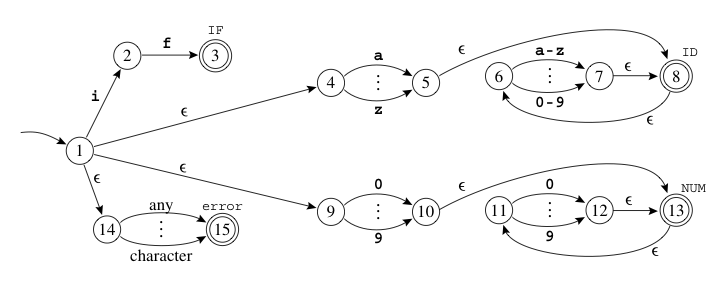#### 概念

1. $$S$$ 是识别器的有限状态集，以及一个错误状态$$S_e$$
2. $$\sum$$是识别器使用的有限字母表。通常，$$\sum$$ 是转移图中边的标签合集
3. $$\delta(s, c)$$是识别器的转移函数。它将每个状态 $$s \in S$$和每个字符$$c \in \sum$$映射到下一个状态。在状态$$s_i$$遇到输入字符$$c$$，FA将采取$$s_i \rightarrow^c \delta(s_i, c)$$
4. $$s_0 \in S$$ 是制定的起始状态
5. $$S_A$$ 是接受状态的集合，$$S_A \subseteq S$$，通常在转移表中为双层圆圈

NFA是不确定有穷自动机（Nondeterministic Finite Automata）
DFA是确定有穷自动机（deterministic finite automaton ）
正则表达式

#### 区别

NFA和DFA最大的区别就是NFA允许输入$$\epsilon$$进行转移，其状态对同一字符输入有可能多种转移

#### 子集构造

###### 1.DFA的状态集

1. edge
$$edge(s, c)$$表示从状态$$s$$沿着字符c可以到达的所有NFA状态的集合
2. closoure(闭包)
对于状态集合$$S$$，$$clousre(S)$$是从$$S$$状态触发，无需接受任何字符，只沿$$\epsilon$$边就可以到达的状态组成的集合。求闭包的算法如下

$$\begin{matrix} T \leftarrow S \\ repeat & T^{'} \leftarrow T \\ & T \leftarrow T^{'} \cup (\cup_{s \in T^{'}}edge(s, \epsilon )) \tag{公式1} \\ until & T = T^{'}\\ \end{matrix}$$

###### 2.起始项

$$DFA\_s_0 = closure( NFA \_s_0 ) \tag{公式3}$$

###### 3.例子起始项
$$NFA \_s_0 = { S_{} }$$
$$DFA\_s_0 = closure( NFA \_s_0 ) = closure({ S_{} } )$$

递推过程#### 其他

公式2 支持分配律$$DFAedge(d, c) = closure(\cup_{s \in d}edge(s, c)) = \cup_{s \in d}(closure(edge(s, c)))$$